April 7, 2020

## What do you need to know?

• Computational Thinking
• Decomposition
• Pattern Recognition
• Abstraction
• Algorithm Design

## Key ideas

• Loops: for(){}, while(){}
• Conditional: if(){}, if(){} else {}

### Practical tools

• Turtle graphics
• Normal graphics: plot(), segments()
• Reading DNA sequences read.fasta()

## Lessons from Homework

• Do it manually first
• Write in R and test
• Be careful with the syntax
• if(){} has two parts: condition and command
• Do not confuse index and content
• Try again and again

## Homework is essential

• It is the only way to practice
• It is the only way to learn
• It gives me feedback on your learning
• It will make you a better professional
• It prepares you for the exam

## Exams

• This years exams will be different from other years
• We need to be sure that each student gets the same chances
• We need to be sure that each answer is personal to the student
• We will be very strict with ethics

## DNA has two strands

FASTA files show only one strand

We call it the forward strand

The other strand is not shown, but can be calculated

We call it the reverse strand

## Calculating the reverse strand

According to Watson & Crick, the reverse strand is complementary

• Change a by t and vice-versa
• Change c by g and vice-versa

Moreover, the reverse strand is reversed

• 5’ becomes 3’ and vice-versa
• i.e. we have to read it backwards
reverse_complement <- function(dna) {
for(i in 1:length(dna)) {
j <- length(dna) - i + 1
if(dna[i]=='a') {
} else if(dna[i]=='c') {
} else if(dna[i]=='g') {
} else if(dna[i]=='t') {
}
}
}

Warning
There is something wrong here.

## Create a vector before using it

We cannot use answer[i] if the vector answer does not exist

It has to be created before

We can create answer as a vector of NA values

answer <- rep(NA, size)

We have the new vector, but we don’t know yet the values

## We need to know the vector’s size

We can create answer with this command

answer <- rep(NA, size)

For this, we need to know the size of the new vector

In this case it must be the same size as the dna vector

(because opposite strands have the same length)

reverse_complement <- function(dna) {
for(i in 1:length(dna)) {
j <- length(dna) - i + 1
if(dna[j]=='a') {
} else if(dna[j]=='c') {
} else if(dna[j]=='g') {
} else if(dna[j]=='t') {
}
}
}

This is the correct version

## Result

dna <-c('t','a','a','c','g','t')
dna
 "t" "a" "a" "c" "g" "t"
reverse_complement(dna)
 "a" "c" "g" "t" "t" "a"

## What happens if we use the reverse strand

Will GC–content change?

Will GC–skew change?

• GC–content does not change
• Because G+C does not change
• GC–skew changes sign
• Because G-C changes sign
• Because each C becomes a G and vice-versa

## Statistics in parts of the genome

Local statistics look only through a small window

A Window is a part of the genome, from an initial position, and with a fixed size

For example, we can analyze a region of 500 bp starting at position 3000 of the genome

## Looking at the window

The function seq() makes numeric sequences

window <- dna[seq(from=3000, length.out=500)]

In the general case, we can write

window <- dna[seq(from=start, length.out=size)]

## Remember the seq() function

seq(from, to, by, length.out)

All inputs are optional

Choose carefully which ones to use

## Examples of seq()

seq(from=5, to=14)
   5  6  7  8  9 10 11 12 13 14
seq(from=5, length.out=10)
   5  6  7  8  9 10 11 12 13 14
seq(from=5, to=30, by=3)
  5  8 11 14 17 20 23 26 29

## Evaluate gc_skew() on different parts of the genome

We traverse the genome with several windows of fixed size

Each window starts after the end of the previous one

In other words, the windows do not overlap

## Create a vector with the window’s positions

We start at the first DNA position, until the last

Be careful to not step out of DNA

win_pos <- seq(from=1, to=length(dna)-size, by=size)

Now we apply gc_skew() to each element of win_pos

The result depends on window’s size

## Result for size=1E5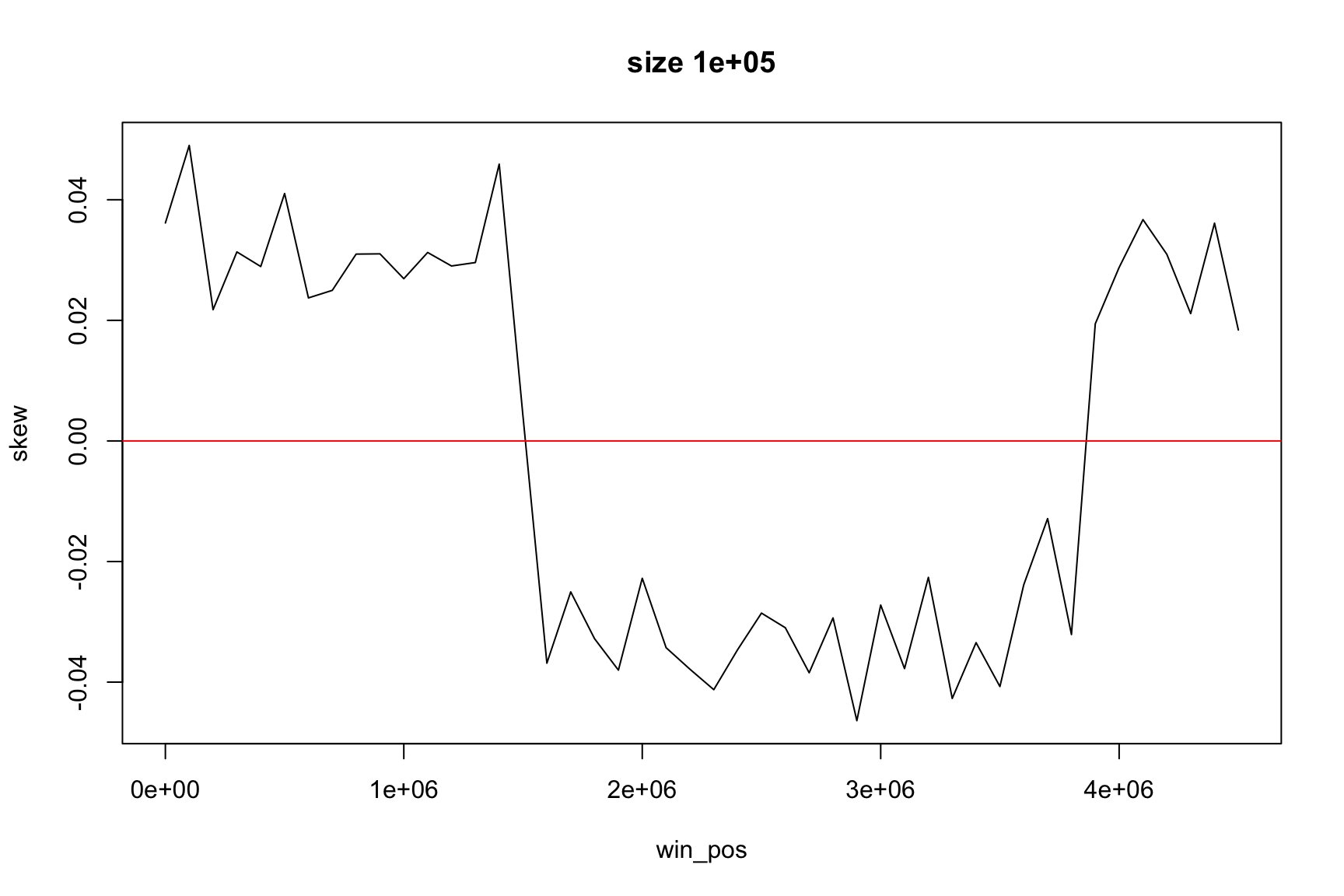## Result for size=1E4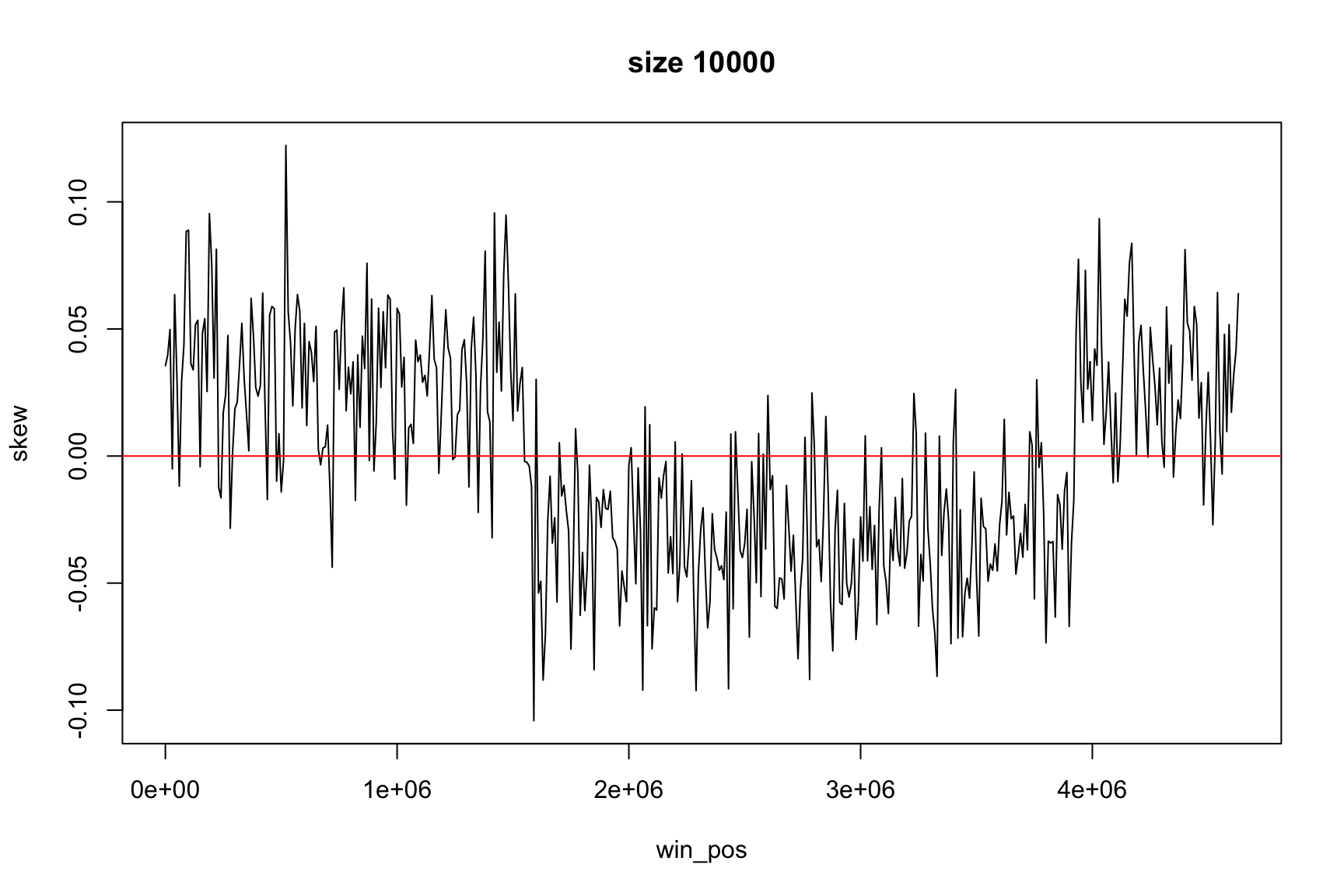## Result for size=1E3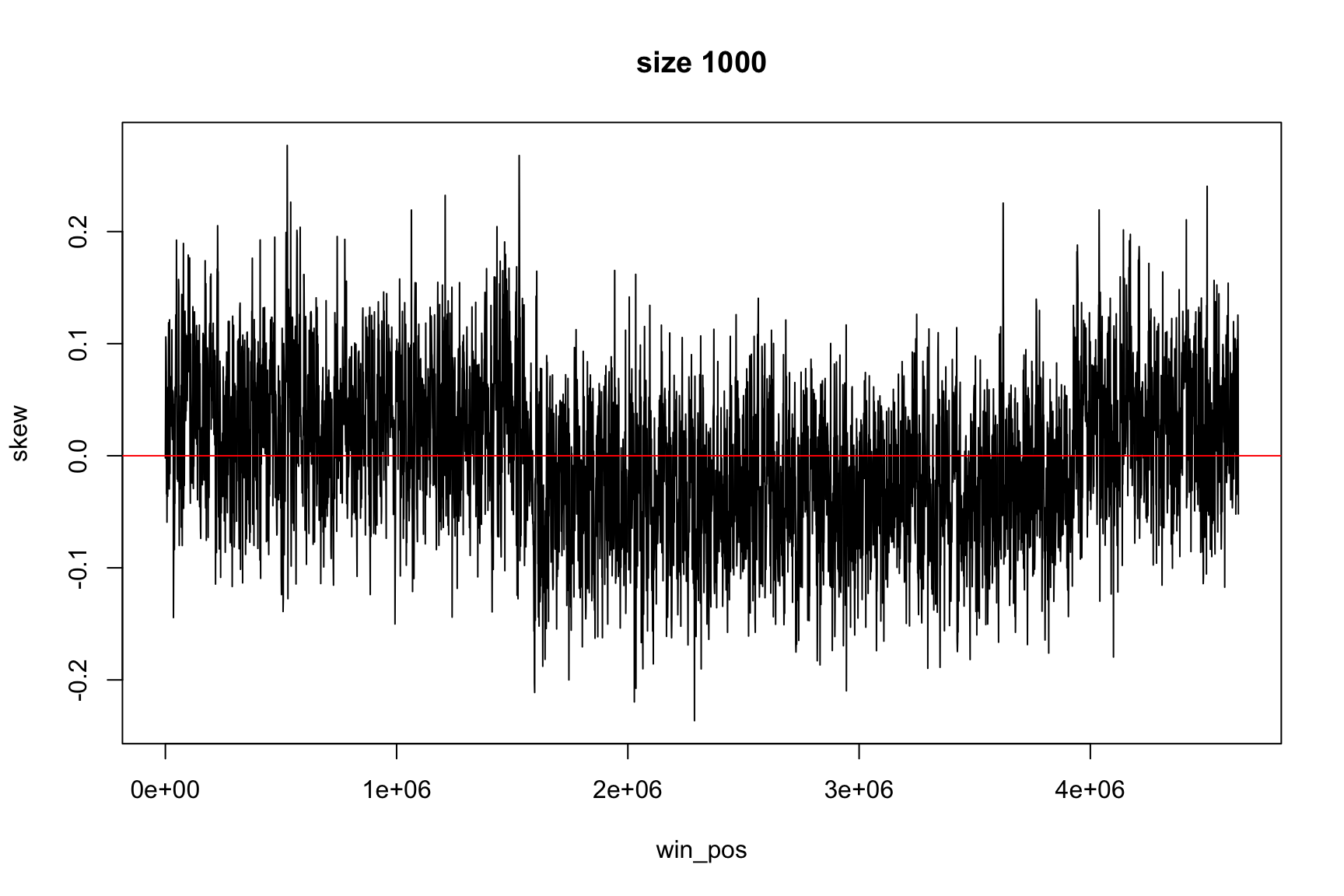## Result for size=1E2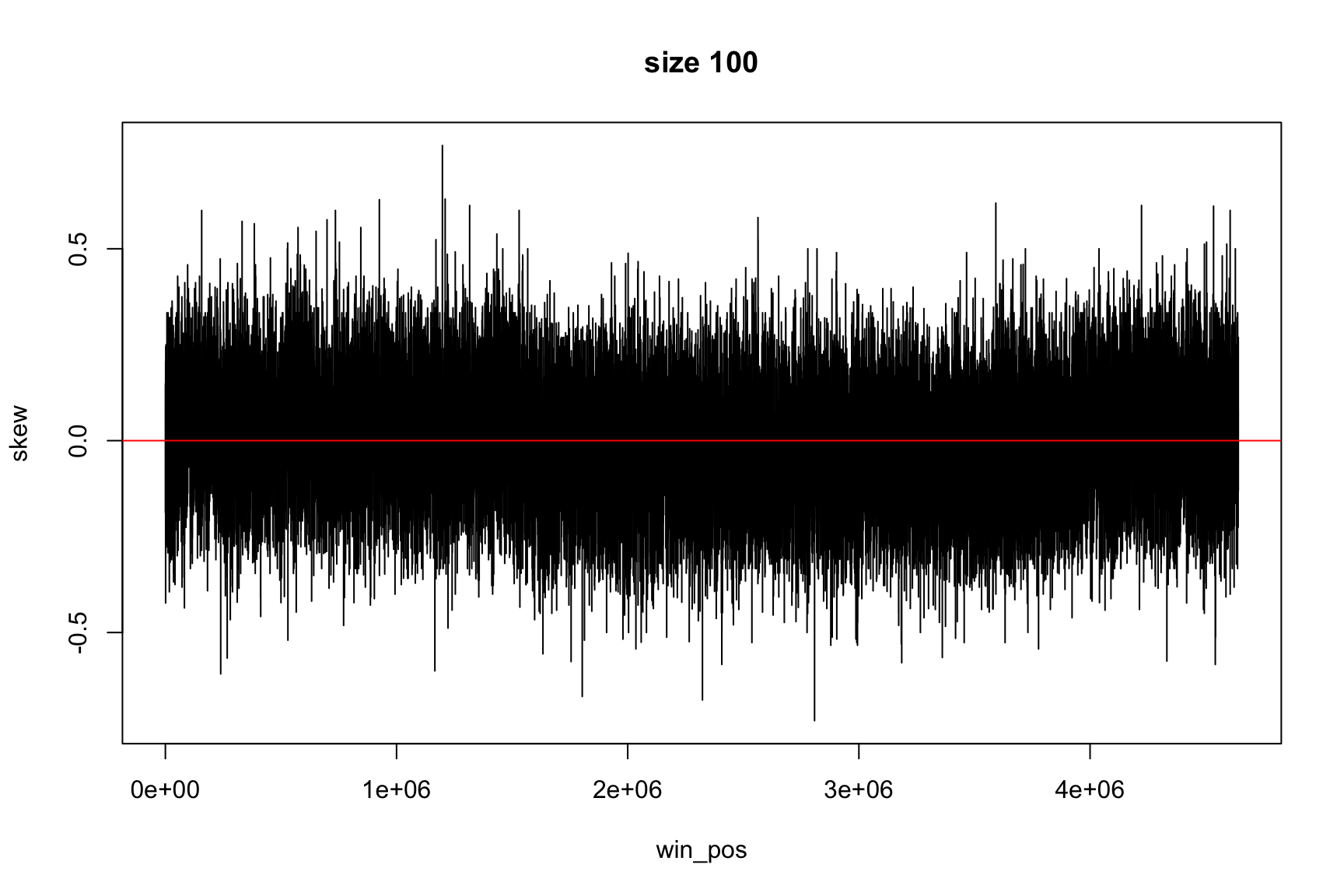## Finding replication origin using GC skew

The relation between %G and %C is not constant over all the genome

In some genome regions there are more G than C

We can evaluate this using the GC skew $\frac{G-C}{G+C}$

## DNA replication

There is a richness of G over C and T over A in the leading strand

(and vice versa for the lagging strand)

GC skew changes sign at the boundaries of the two replichores

This corresponds to DNA replication origin or terminus

## DNA replication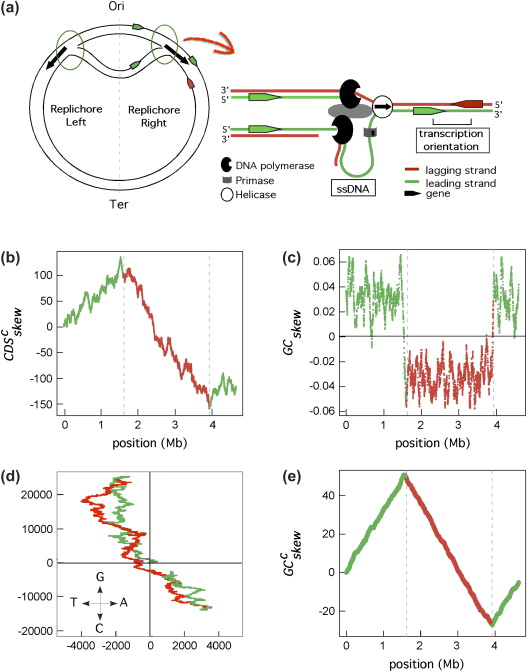Marie Touchon, Eduardo P.C. Rocha, (2008), From GC skews to wavelets: A gentle guide to the analysis of compositional asymmetries in genomic data,
Biochimie, Volume 90, Issue 4,

## Finding the origin

• The GC skew is positive in one part of chromosome
• And negative on the other, on average

The reason for this asymmetry is the DNA replication process

The origin of replication is in one of the sign changing places

## How can we find the change of sign

We want to write a program so we can find Ori on many different genomes

• Are both replichores of the same size?
• If they are different, what is the biological reason?
• Is there some insertion?
• What are the extra genes? What do they do?

## Finding the change of sign is not so easy

The values can change a lot between a window and the next one

skew data is noisy. A lot of small random changes

## There is an easier way to find Ori

Instead of looking directly at skew, we can look at accumulative skew

$\text{acc_skew}[i]=\text{skew}[i]+\text{skew}[i-1]+\cdots+\text{skew}$

Now, instead of the change of sign, we look for the minimum and maximum (Why?)

## Now it is easier to see the change of sign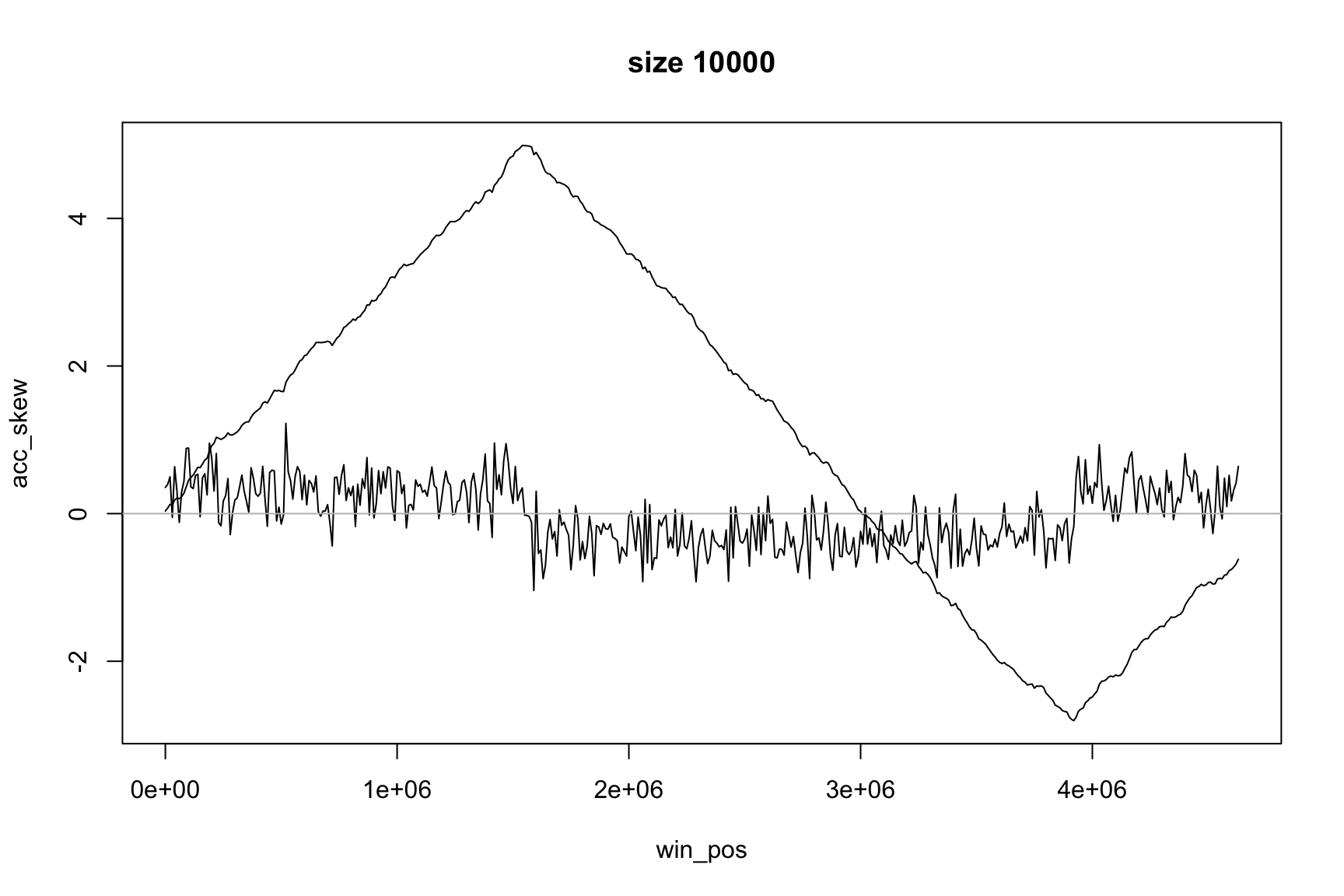## The dictionary says

accumulative
§ increasing, accumulative, growing, mounting; collective, aggregate, amassed.
§ the accumulative effect of two years of drought
§ the effects of pollution are accumulative

## Accumulative sum

Everyday you spend some money

How much money you have each day?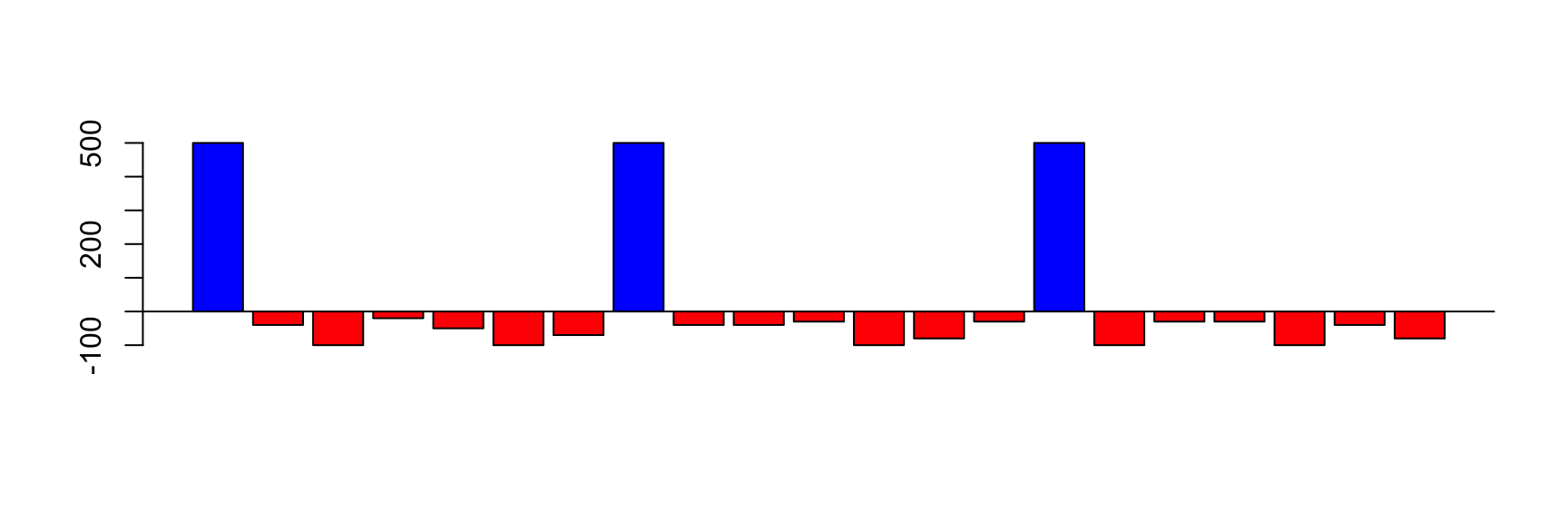## Accumulative Sum: daily balance

The income can be positive (salary) or negative (expenses)

The balance on first day is your initial money

The balance of today is the balance of yesterday plus the income of today

balance[today] <- balance[yesterday] + income[today]

## balance and income are vectors

Since they have different values every day, we represent the income on day i by income[i]

We also represent the balance on day i by balance[i]

The vector income has the values for all days

The element income[i] has the value for a single day

## Today and yesterday

We will see several “days”, represented by i

It is useful to think that

• i represents “today”
• i-1 represents “yesterday”

## If we know income, we can calculate balance

The balance on first day is your initial money

balance <- income

The balance of today is the balance of yesterday plus the income of today

for(i in 2:length(income)) {
balance[i] <- balance[i-1] + income[i]
}

## Balance looks like this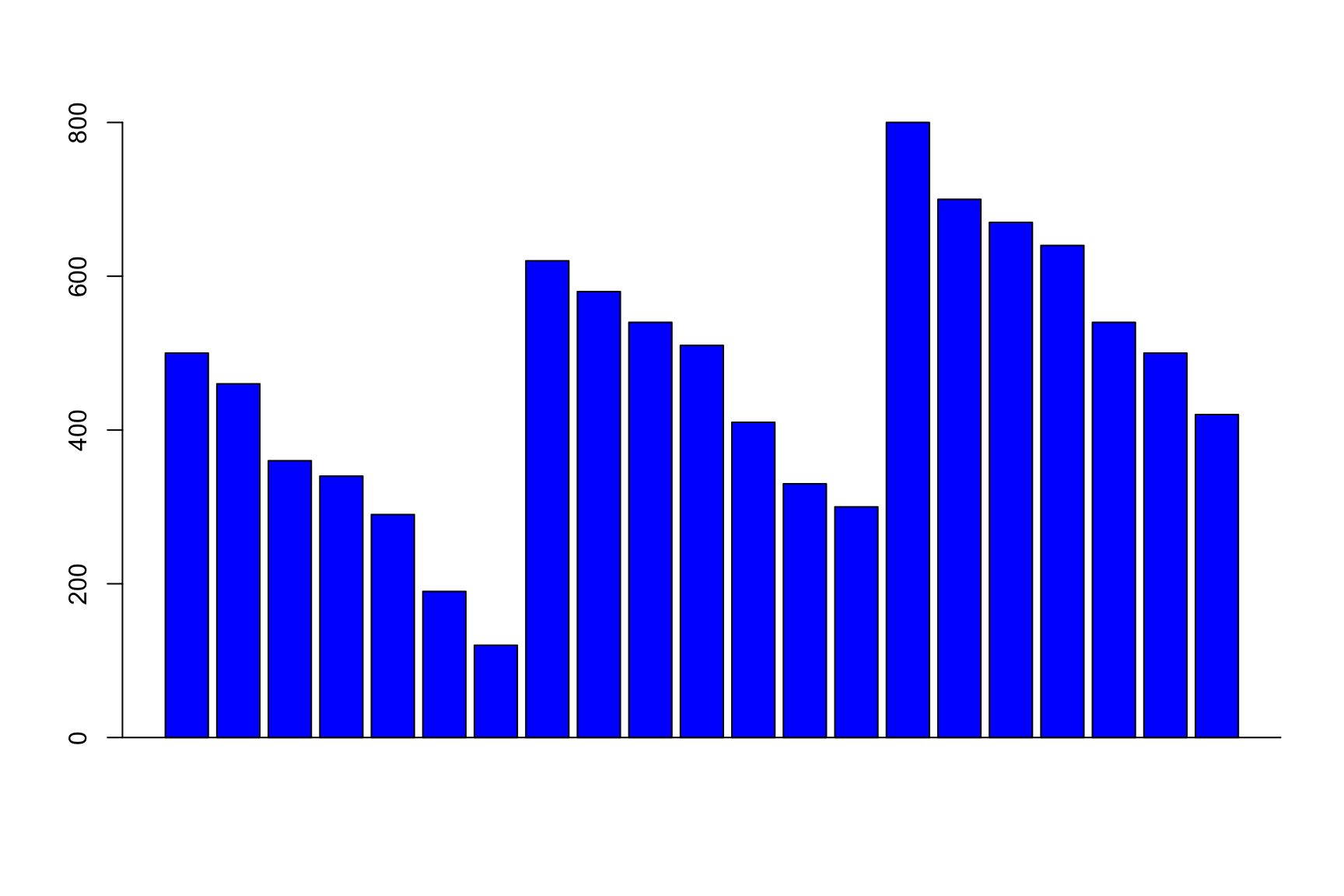## The same idea applies to GC-skew

The GC skew in each window is like the income in each day

And we want to see the “balance”: accumulative skew

acc_skew <- skew
for(i in 2:length(skew)) {
acc_skew[i] <- acc_skew[i-1] + skew[i]
}

Same pattern, same solution

Can you write this as a function?

## Now max & min show the change of sign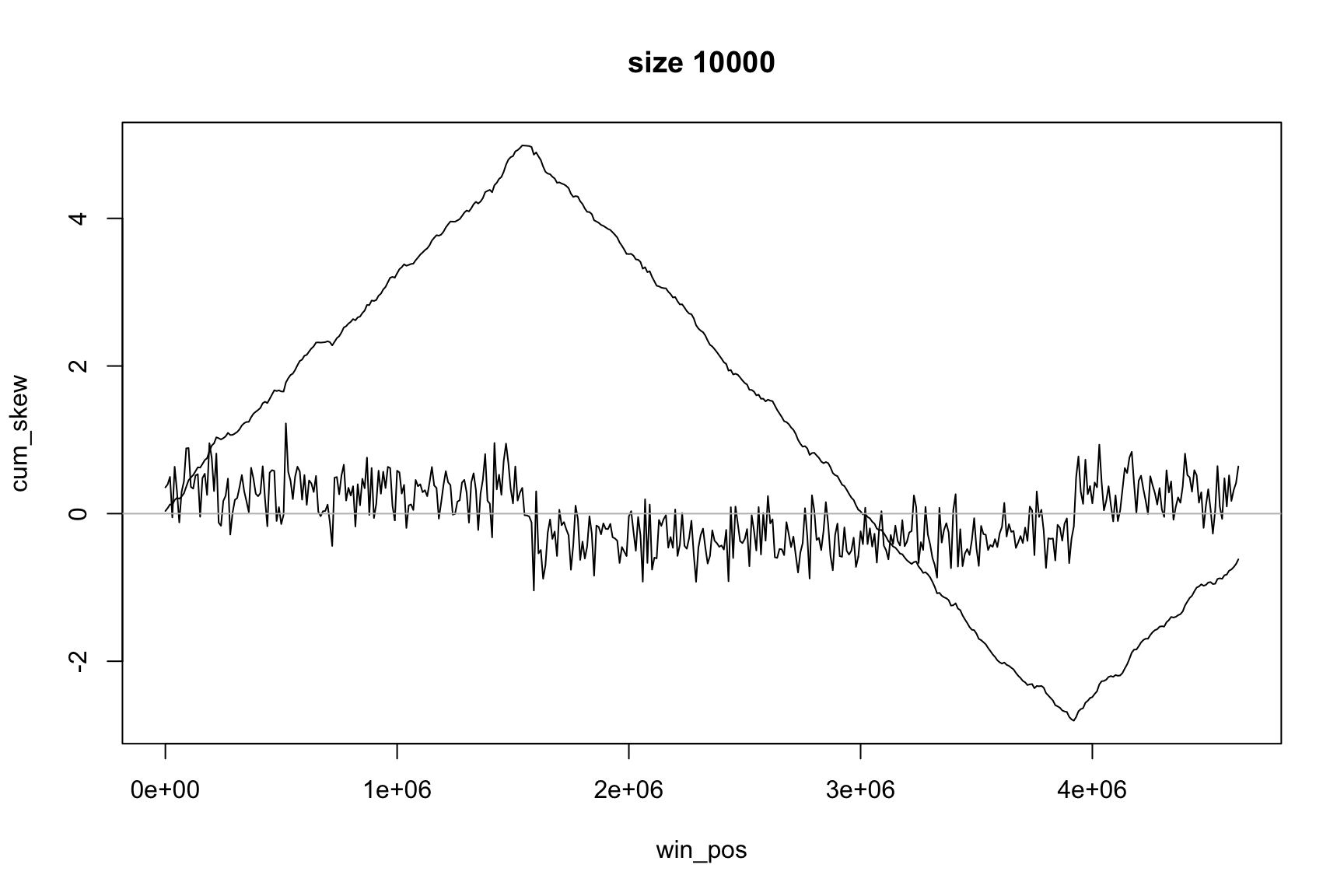## Position of the maximum

The maximum value of acc_skew is

max(acc_skew)
 4.9877

It is not really important

We are looking for position, not value of maximum

## Position of the maximum

This is the index of the maximum in the acc_skew vector

which.max(acc_skew)
 155

This is always an integer number, between 1 and length(acc_skew)

It is not the position in the genome

## Position of the maximum

Since acc_skew vector has the same size as win_pos, we can find the Ori position with this

win_pos[which.max(acc_skew)]
 1540001

win_pos contains the genome positions of each window

This code gives us the position of the window with maximum GC-skew

## Locating origin depends on window size

Ori is located in the interval between

• win_pos[which.max(acc_skew)]
• win_pos[which.max(acc_skew)] + size

## Skew and Accumulative skew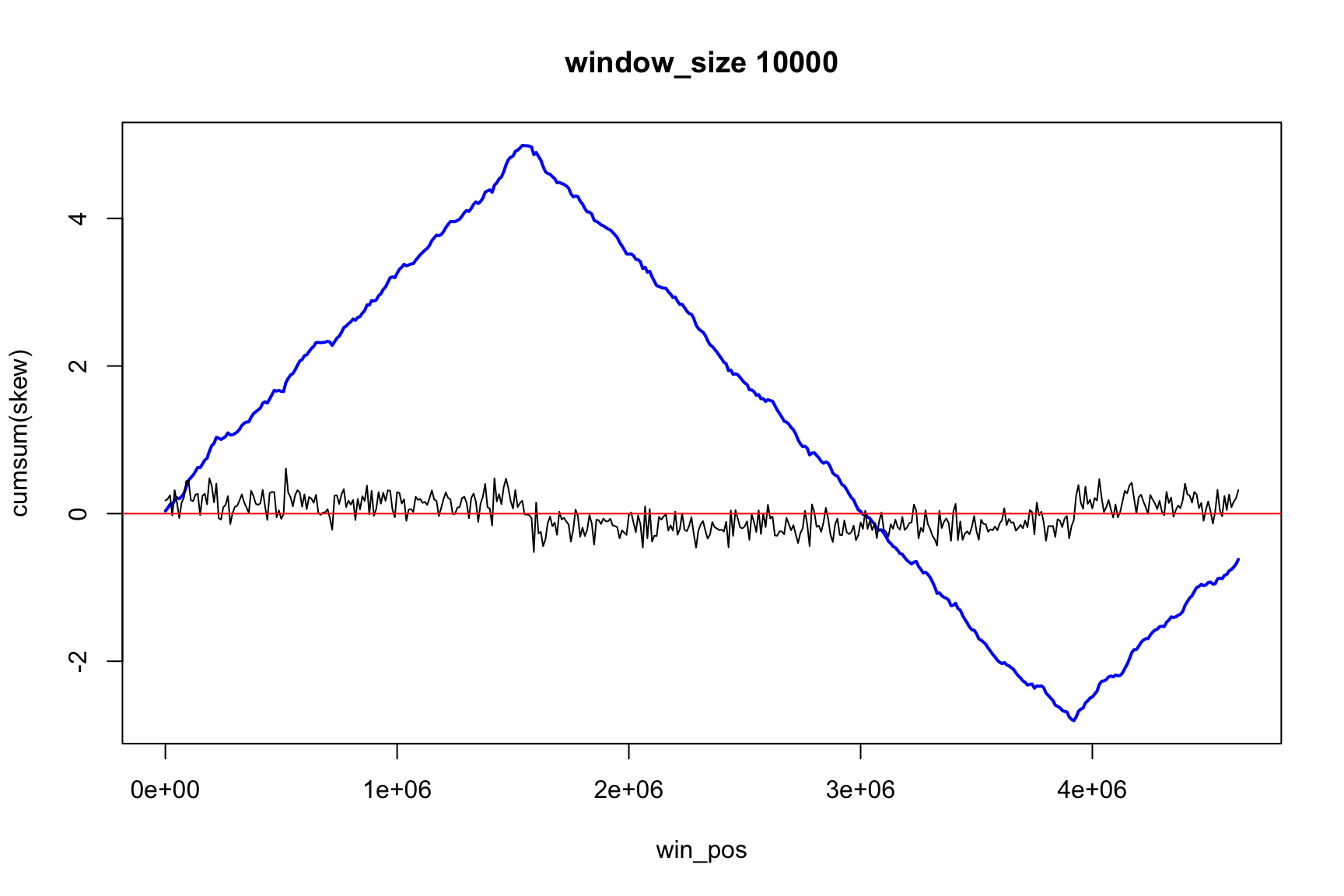## Skew and Accumulative skew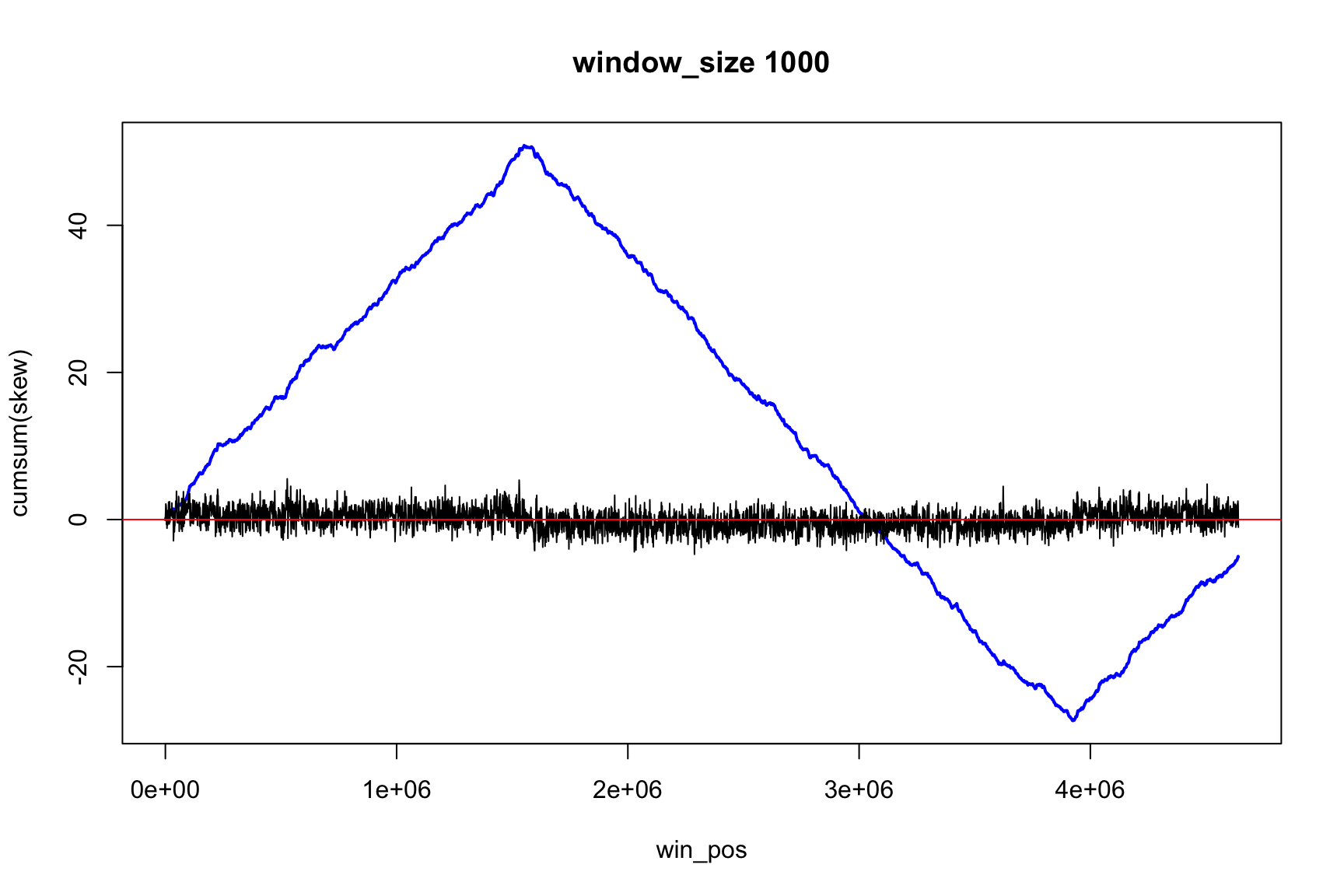## Skew and Accumulative skew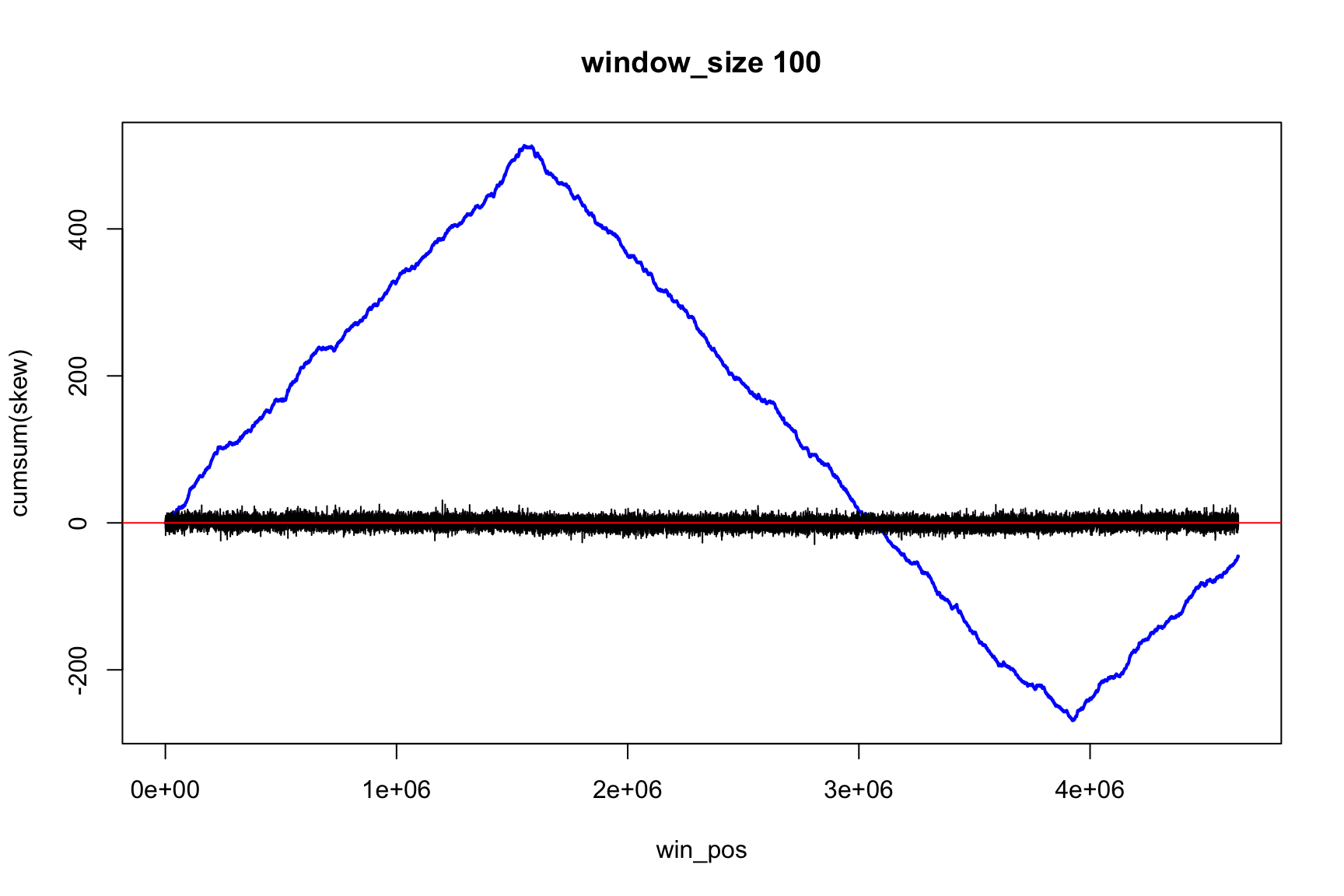## Skew and Accumulative skew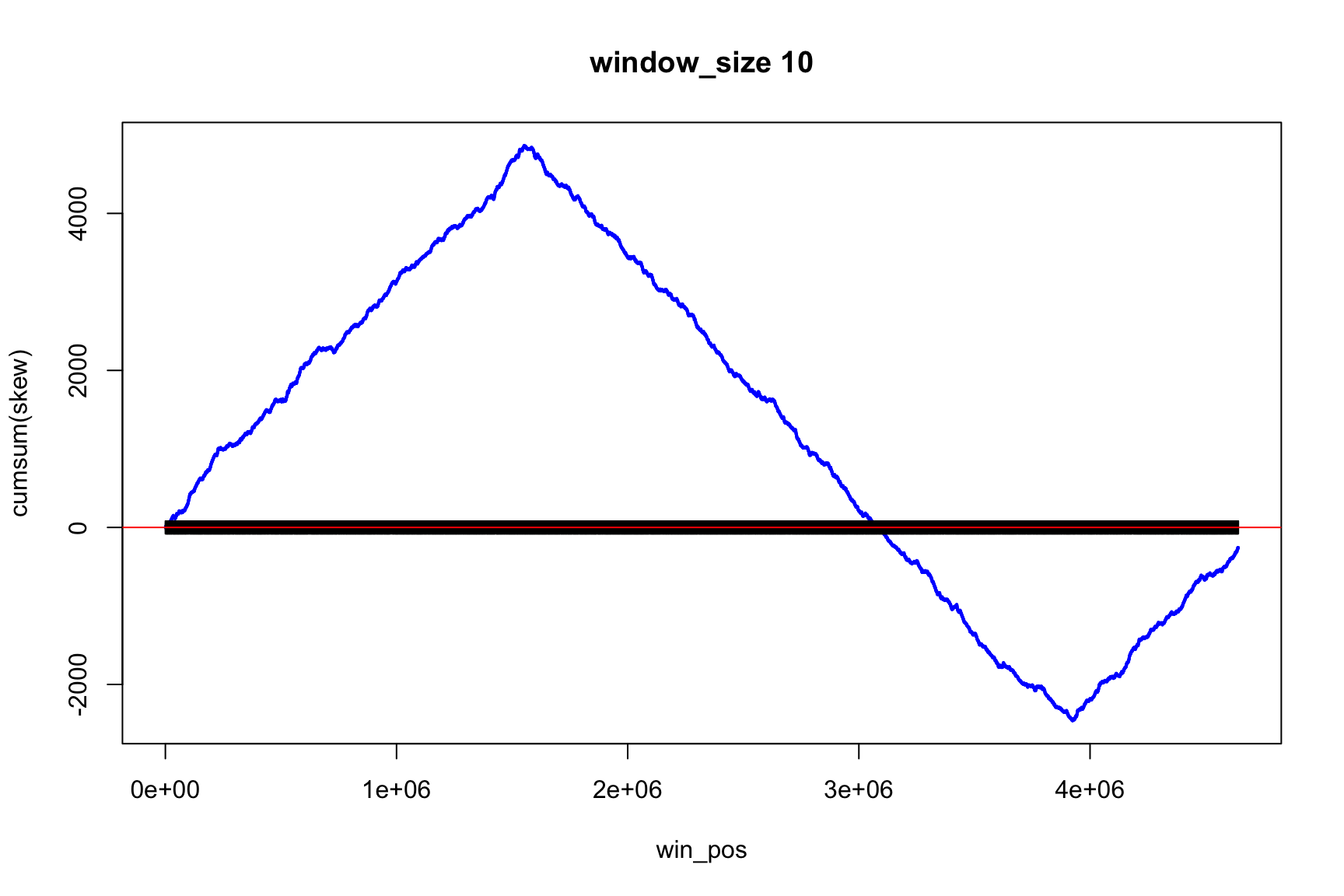## skew changes fast, acc_skew changes slow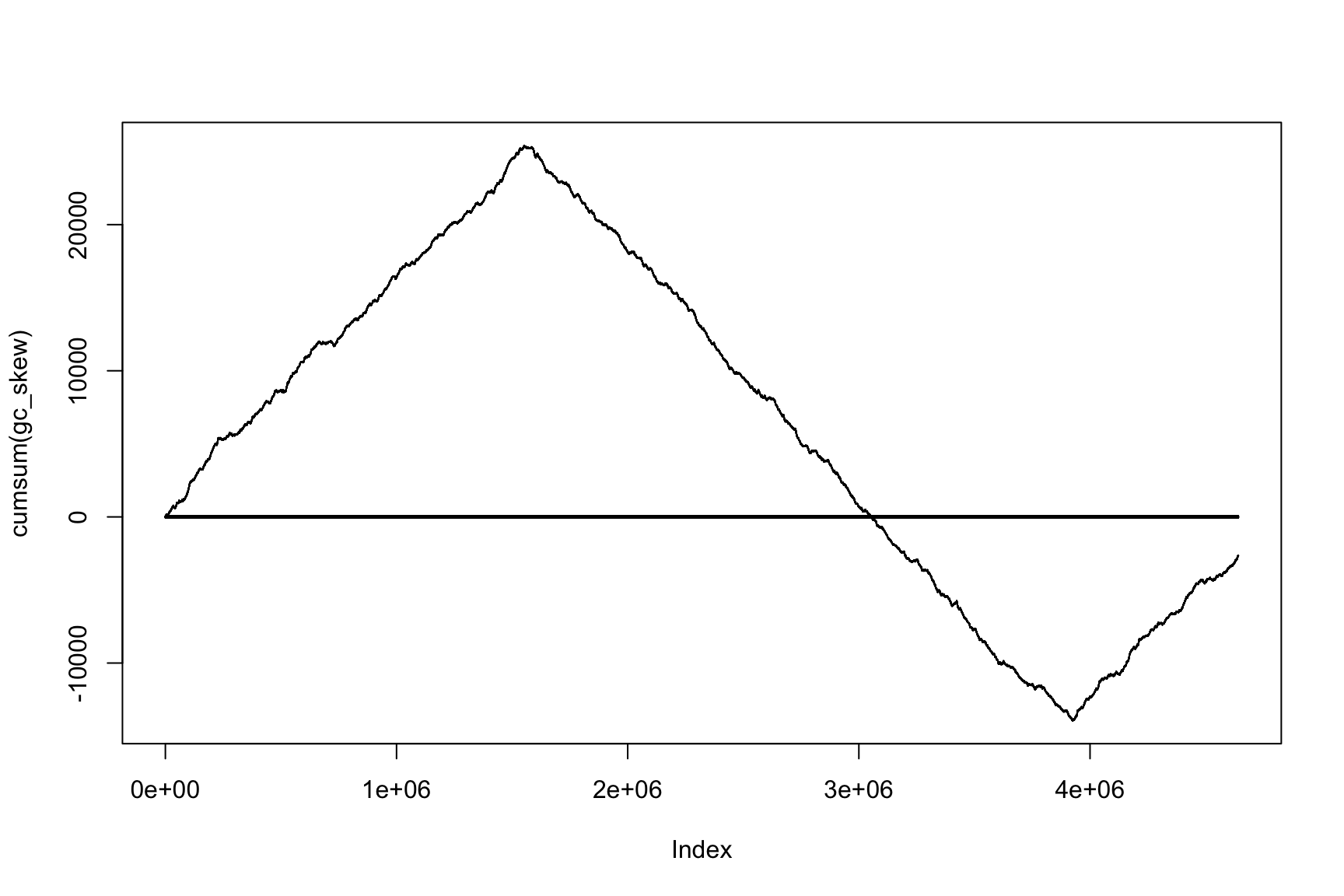## Accumulative sums are useful

• If we know the income, we can get balance
• If we know the skew, we can get acc_skew
• If we know the change, we can know the state